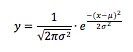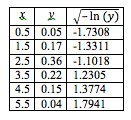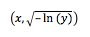## Tuesday, May 31, 2011

### Fitting a normal curve

I show my students histograms of more or less normally distributed real-life data. I have found it difficult, though, to get a Normal curve that fits nicely on top of the histogram. Is there a way to do a best-fit regression in this situation? I looked around and can't find one, so here's a procedure I came up with. I'm not sure if it's the best possible fit, but it's a good fit.

Using the fact that a normal distribution is given by the equationyou can work backwards to see how to make the data linear. That is,is a linear transformation of normally distributed data. Here is a histogram.I used the midpoint of each bin as the x data, and then I transformed the y data as described.The one trick is that, for x values below the mean, the transformed y data points need to be negative. That can create a little ambiguity for the middle bin, but it's not too hard to tell here that 2.5 is a little below the mean. If in doubt, try both. I ran a linear regression onand found thatThis can be transformed back intowhich is almost ready to graph. All it's missing is the leading coefficient. A little work shows that the standard deviation is 0.88, and therefore the final equation isHere's the histogram, with the overlaid normal curve, which does not fit especially well.However, this shows that this real-life data does not exactly follow a normal distribution, since this is about as well as we could hope it would fit.